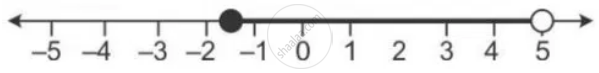Share

# Solve the Following Inequation and Graph the Solution Set on the Number Line: 2x – 3 < X + 2 ≤ 3x + 5, X ∈ R. - Mathematics

Course

#### Question

Solve the following inequation and graph the solution set on the number line:
2x – 3 < x + 2 ≤ 3x + 5, x ∈ R.

#### Solution

2x – 3 < x + 2 ≤ 3x + 5
2x – 3 < x + 2 and x + 2 ≤ 3x + 5
x < 5 and -3 ≤ 2x
x < 5 and -1.5 ≤ x
Solution set = {-1.5 ≤ x < 5}
The solution set can be graphed on the number line as:Is there an error in this question or solution?